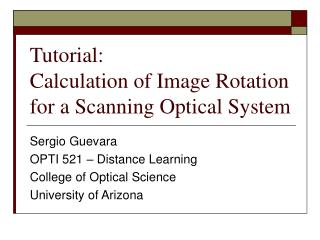DownloadDownload PresentationTutorial: Calculation of Image Rotation for a Scanning Optical System

# Tutorial: Calculation of Image Rotation for a Scanning Optical System

Télécharger la présentation## Tutorial: Calculation of Image Rotation for a Scanning Optical System

- - - - - - - - - - - - - - - - - - - - - - - - - - - E N D - - - - - - - - - - - - - - - - - - - - - - - - - - -
##### Presentation Transcript

1. Tutorial: Calculation of Image Rotation for a Scanning Optical System Sergio Guevara OPTI 521 – Distance Learning College of Optical Science University of Arizona

2. Brief Overview of Scanning Optical Systems • Uses: • Image Scanning • IR Imaging Systems • Laser Scanning • Laser Printers • Laser Projectors • Microvision’s SHOW Projector • Artifacts • Image Distortion • Image Rotation Figure from US Patent #4,106,845

3. Steps to Analyze Image Rotation • Sketch the system including: • Mirror locations • Mirrors’ axis of rotation. • Input beam. • Image plane. • Choose a global coordinate system. • Define all objects in the global coordinate system. • Define mirror matrices for mirrors in global coordinate system. • Define axis of rotation for mirrors in global coordinate system. • Find image for non-rotated case. • Add rotation to mirrors. • Find image in rotated case. • Project rotated and non-rotated images onto image plane. • Compare the projected images of the rotated case with the non-rotated case to determine rotation of image.

4. Sketch the System Top View Top View Figure from US Patent #4,106,845

5. Sketch the System Front View Right View Figure from US Patent #4,106,845

6. Choose Global Coordinate System Top View Right View

7. Define Input Beam & Image Planein Global Coordinates • Input Beam is Parallel to Z-axis. • Image Plane lies on the X-Z plane.

8. Define Mirrors in Global Coordinates • Find Mirror’s Normal Unit Vectors • Mirror 1 normal unit vector: • Mirror 2 normal unit vector: • Create Mirror Matrices • Mirror 1 Matrix: • Mirror 2 Matrix:

9. Define Rotation of Mirrorsin Global Coordinates • Mirror Rotation • Rotation Matrices • Mirror 1 Rotation Matrix:(where α is the angle of rotation) • Mirror 2 Rotation Matrix:(where β is the angle of rotation)

10. System Model • Input thru Mirror • For no image rotation • Projection onto Image Plane

11. Calculation of Rotation • Is a comparison of the initial image with no mirror rotation to an image with mirror rotation. • Use the definition of the cross product: • Rotation Equation:

12. Calculations

13. Questions?

14. Rotation Matrices via Euler Parameters • Euler Parameters where the axis of rotation is a unit vector, , and the angle of rotation about that axis is, . • Rotation Matrix in Einstein Notation where δij is the Kronecker delta, and εijk is the permutation symbol.

15. Further Resources • How to perform mirror rotations in Zemax • http://www.zemax.com/kb/articles/25/1/How-To-Model-a-Scanning-Mirror/Page1.html • Euler Parameters • http://mathworld.wolfram.com/EulerParameters.html • Line onto Plane Projection • http://www.euclideanspace.com/maths/geometry/elements/plane/lineOnPlane/index.htm • http://www.euclideanspace.com/maths/geometry/elements/line/projections/index.htm.